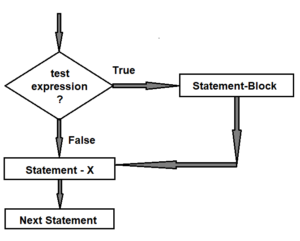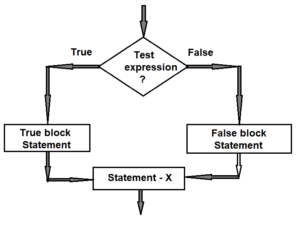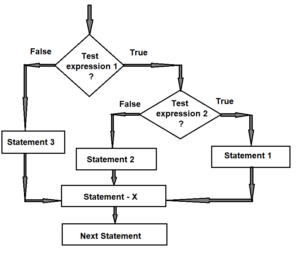# Conditional statement in C | Conditional statement

In this article Conditional statement in C we give the information about the if statement is a powerful decision-making statement and is used to control the flow of statements.

#### Conditional statement in C:

Introduction:

We have seen that the C program is a set of statements that are usually executed in the order in which they appear. This happens when no duplication of any frequency or specific calculation is required.

However, in practice, we have many situations where we have to change the order of execution of statements based on certain conditions or repeat the set of statements until certain conditions are met. This involves making some sort of decision to see if a particular condition has occurred and then instructing the computer to execute certain statements accordingly.

C has the ability to make decisions based on the following statements:

1. If the statement
2. Switch statements
3. Conditional operator statement
4. Go to the statement.

These statements are known as decision making statements. Since these statements ‘control’ the flow of execution, they are also called control statements.

#### Conditional statement:

The if statement is a powerful decision-making statement and is used to control the flow of statements.

Statements can be applied in a variety of forms depending on the complexity of the situation being tested.

The different forms are:

1. Simple if statement
2. if…..else statement
3. Nested if…..else statement

#### 1.Simple if Statement:

Syntax:

if (test expression)

{

statement-block;

}

statement-x;

A ‘statement-block’ can be a single statement or a set of statements. If the text expression is true, the statement-block will be executed; otherwise the statement-block will be omitted and the execution will go to statement-x.#### Flowchart of Simple if Statement

Program:

void main()

{

int age = 20;.

clrscr();

if (age>=58)

{

printf(“\n Person is retired “);

}

Printf(“\n Have a nice Day!”);

getch();

}

OutPut:

Have a nice Day!

#### 2.The if…else statement:

The if…else statement is developed by  the simple if statement.

Syntax:

if (test expression)

{

true-block statements;

}

else

{

false-block statements;

}

statement-x;

If the test expression is true then the truth-block statement is executed; Otherwise, false-block statements is executed. In any case, either true-block or false-block will be executed, not both at a time.#### Flowchart of if else Statement

Progarm:

// Odd-Even Number

void  main()

{

int number;

clrscr();

printf(“\n Enter an integer: “);

scanf(“%d”, &number);

// True if the number is divisible by 2

if(number % 2 == 0)

printf(“%d is even.”, number);

else

printf(“%d is odd.”, number);

getch();

}

OutPut:

Enter an integer: 10

10 is even.

#### 3.Nested if … else statement:

Nested if … else statement

When a series of decisions is involved. If you have to use more than possibility then Nested if …else will have used.

Syntax:

if (test expression 1)

{

if(test expression 2)

{

statement(s) – 1;

}

else

{

statement(s) – 2;

}

}

else

{

statement(s) – 3;

}

statement(s) – x;#### Flowchart of nested if else Statement

// Program to relate two integers using =, > or <

void  main()

{

int number1, number2;

printf(“Enter two integers: “);

scanf(“%d %d”, &number1, &number2);

if(number1 == number2)               //checks if two integers are equal.

{

printf(“Result: %d = %d”,number1,number2);

}

else if (number1 > number2)         //checks if number1 is greater than number2.

{

printf(“Result: %d > %d”, number1, number2);

}

else                                // if both test expression is false

{

printf(“Result: %d < %d”,number1, number2);

}

getch();

}

Output:

Enter two integers: 12

23

Result: 12 < 23

#### Some More:

POP- Introduction to Programming Using ‘C’

DS – Data structure Using C

OOP – Object Oriented Programming

Java Programming

DBMS – Database Management System

RDBMS – Relational Database Management System